# Freq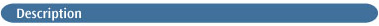This calculation type keyword computes force constants and the resulting vibrational frequencies. Intensities are also computed. By default, the force constants are determined analytically if possible (for RHF, UHF, MP2, CIS, all DFT methods, and CASSCF), by single numerical differentiation for methods for which only first derivatives are available (MP3, MP4(SDQ), CID, CISD, CCD, QCISD, and all semi-empirical methods), and by double numerical differentiation for those methods for which only energies are available.

Vibrational frequencies are computed by determining the second derivatives of the energy with respect to the Cartesian nuclear coordinates and then transforming to mass-weighted coordinates. This transformation is only valid at a stationary point! Thus, it is meaningless to compute frequencies at any geometry other than a stationary point for the method used for frequency determination.

For example, computing 3-21G frequencies at a STO-3G optimized geometry produces meaningless results. It is also incorrect to compute frequencies for a correlated method using frozen-core at a structure optimized with all electrons correlated, or vice-versa. The recommended practice is to compute frequencies following a previous geometry optimization using the same method. This may be accomplished automatically by specifying both Opt and Freq within the route section for a job.

Note also that the coupled perturbed Hartree-Fock (CPHF) method used in determining analytic frequencies is not physically meaningful if a lower energy wavefunction of the same spin multiplicity exists. Use the Stable keyword to test the stability of Hartree-Fock and DFT wavefunctions.

### FREQUENCY CALCULATION VARIATIONS

When frequencies are done analytically, polarizabilities are also computed automatically; when numerical differentiation is required (or requested with Freq=Numer), polarizabilities must be explicitly requested using the Polar keyword (e.g., QCISD Freq Polar).

The VCD option may be used to compute the vibrational circular dichroism (VCD) intensities in addition to the normal frequency analysis at the Hartree-Fock and DFT levels .

Pre-resonance Raman intensities may be computed by specifying a Raman option, and also including CPHF=RdFreq within the route and specifying the desired frequency in the input file (see the examples for additional information).

Frequency-dependent polarizabilities and hyperpolarizabilities may similarly be computed by including CPHF=RdFreq within the route (subject to their usual availability restrictions).

The keyword Opt=CalcAll requests that analytic second derivatives be done at every point in a geometry optimization. Once the requested optimization has completed all the information necessary for a frequency analysis is available. Therefore, the frequency analysis is performed and the results of the calculation are archived as a frequency job.

You should specify alternative isotopes for frequency jobs using the standard method. However, the ReadIsotopes option is retained for rerunning completed calculations under different conditions (see the examples).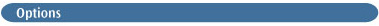VCD
Compute the vibrational circular dichroism (VCD) intensities in addition to the normal frequency analysis . This option is valid for Hartree-Fock and DFT methods. This option also computes optical rotations (see Polar=OptRot).

ROA
Compute the Raman Optical Activity [579, 583, 584]. By default, this is done for the static limit, but the CPHF=RdFreq keyword indicates that one or more incident light frequencies should be read in and used in the electromagnetic perturbations.

Raman
Compute Raman intensities in addition to IR intensities. This is the default for Hartree-Fock. It may be specified for DFT and MP2 calculations in order to produce Raman intensities by numerical differentiation of dipole derivatives with respect to the electric field. For these methods, it is equivalent to NRaman. If CPHF=RdFreq is used, then Raman is equivalent to NNRaman for all methods.

NRaman
Do polarizability derivatives by numerically differentiating the analytic dipole derivatives with respect to an electric field. This is the default for CIS, DFT, and MP2 if Raman is requested but CPHF=RdFreq is not.

NNRaman
Do polarizability derivatives by numerically differentiating the analytic polarizability with respect to nuclear coordinates. This is the default if Raman is requested along with CPHF=RdFreq.

NoRaman
Skips the extra steps required to compute the Raman intensities during Hartree-Fock analytic frequency calculations, saving 10-30% in CPU time.

VibRot
Analyze vibrational-rotational coupling [206,207,208,209,210,211,493,494,495].

Anharmonic
Do numerical differentiation along normal modes to compute zero-point energies, anharmonic frequencies [206,208,209,211,493,494,495,577,578], and anharmonic vibrational-rotational couplings if VibRot is also specified [207,210,212,213,214]. This option is only available for methods with analytic second derivatives: Hartree-Fock, DFT, CIS and MP2.

ReadAnharm
Read an input section with additional parameters for the vibration-rotation coupling and/or anharmonic vibrational analysis (VibRot or Anharmonic options). Available input options are documented following the examples.

ReadFC
Requests that the force constants from a previous frequency calculation be read from the checkpoint file, and the normal mode and thermochemical analysis be repeated, presumably using a different temperature, pressure, or isotopes, at minimal computational cost. Note that since the basis set is read from the checkpoint file, no general basis should be input. If the Raman option was specified in the previous job, then do not specify it again when using this option.

HPModes
Include the high precision format (to five figures) vibrational frequency eigenvectors in the frequency output in addition to the normal three-figure output.

InternalModes
Print normal modes as displacements in redundant internal coordinates. IntModes is a synonym for this option.

Analytic
This specifies that the second derivatives of the energy are to be computed analytically. This option is available only for RHF, UHF, CIS, CASSCF, MP2, and all DFT methods, and it is the default for those cases.

Numerical
This requests that the second derivatives of the energy are to be computed numerically using analytically calculated first derivatives. It can be used with any method for which gradients are available and is the default for those for which gradients but not second derivatives are available. Freq=Numer can be combined with Polar=Numer in one job step.

EnOnly
This requests double numerical differentiation of energies to produce force constants. It is the default and only choice for those methods for which no analytic derivatives are available. EnergyOnly is a synonym for EnOnly.

Cubic
Requests numerical differentiation of analytic second derivatives to produce third derivatives.

Step=N
Specifies the step-size for numerical differentiation to be 0.0001*N (in Angstoms unless Units=Bohr has been specified). If Freq=Numer and Polar=Numer are combined, N also specifies the step-size in the electric field. The default is 0.001 Å for Hartree-Fock and correlated Freq=Numer, 0.005 for GVB and CASSCF Freq=Numer, and 0.01 Å for Freq=EnOnly. For Freq=Anharmonic or Freq=VibRot, the default is 0.025.

Restart
This option restarts a numerical frequency calculation after the last completed geometry (analytic frequency calculations are not restartable). A failed numerical frequency job may be restarted from its checkpoint file by simply repeating the route section of the original job, adding the Restart option to the Freq keyword. No other input is required.

Projected
For a point on a mass-weighted reaction path (IRC), compute the projected frequencies for vibrations perpendicular to the path. For the projection, the gradient is used to compute the tangent to the path. Note that this computation is very sensitive to the accuracy of the structure and the path . Accordingly, the geometry should be specified to at least 5 significant digits. This computation is not meaningful at a minimum.

HinderedRotor
Requests the identification of internal rotation modes during the harmonic vibrational analysis . If any normal modes are identified as internal rotation, hindered or free, the thermodynamic functions are corrected. The identification of the rotating groups is made possible by the use of redundant internal coordinates. Thus, redundant internal coordinates must be used for the HinderedRotor option to function properly. Because some structures, such as transition states, may have a specific bonding pattern not automatically recognized, the set of redundant internal coordinates may need to be altered via the Geom=Modify keyword.

If the force constants are available on a previously generated checkpoint file, additional vibrational/internal rotation analyses may be performed by specifying Freq=(ReadFC, HinderedRotor). Since Opt=CalcAll automatically performs a vibrational analysis on the optimized structure, Opt=(CalcAll, HinderedRotor) may also be used.

ModRedundant
Read-in modifications to redundant internal coordinates (i.e., for use with InternalModes). Note that the same coordinates are used for both optimization and normal mode analysis in an Opt Freq, for which this is the same as Opt=ModRedundant. See the discussion of the Opt keyword for details on the input format.

ReadIsotopes
Specify alternate temperature, pressure, and/or isotopes (the defaults are 298.15 K, 1 atmosphere, and the most abundant isotopes). By default, this option uses temperature, pressure, and scale factor specified in the route section. Alternatively, this information can appear in a separate input section having the format:

```temp pressure [scale]                                                                   Must be real numbers.
isotope mass for atom 1
isotope mass for atom 2
...
isotope mass for atom n ```

where temp, pressure, and scale are the desired temperature, pressure, and an optional scale factor for frequency data when used for thermochemical analysis (the default is unscaled). The remaining lines hold the isotope masses for the various atoms in the molecule, arranged in the same order as they appeared in the molecule specification section. If integers are used to specify the atomic masses, the program will automatically use the corresponding actual exact isotopic mass (e.g., 18 specifies O18, and Gaussian uses the value 17.99916).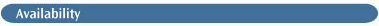Analytic frequencies are available for the HF, DFT, MP2, CIS and CASSCF methods. Numerical frequencies are available for MP3, MP4(SDQ), CID, CISD, CCD, CCSD and QCISD. ROA is available for HF and DFT methods.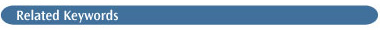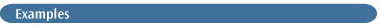Frequency Output. The basic components of the output from a frequency calculation are discussed in detail in chapter 4 of Exploring Chemistry with Electronic Structure Methods .

You may be surprised to see output that looks like it belongs to a geometry optimization at the beginning of a frequency job:

```GradGradGradGradGradGradGradGradGradGradGradGradGradGradGrad
Berny optimization.
Initialization pass. ```

Link 103, which performs geometry optimizations, is executed at the beginning and end of all frequency calculations. This is done so that the quadratic optimization step can be computed using the correct second derivatives. Occasionally an optimization will complete according to the normal criterion using the approximate Hessian matrix, but the step size is actually larger than the convergence criterion when the correct second derivatives are used. The next step is printed at the end of a frequency calculation so that such problems can be identified. If you think this concern is applicable, use Opt=CalcAll instead of Freq in the route section of the job, which will complete the optimization if the geometry is determined not to have fully converged (usually, given the full second derivative matrix near a stationary point, only one additional optimization step is needed), and will automatically perform a frequency analysis at the final structure.

Specifying #P in the route section produces some additional output for frequency calculations. Of most importance are the polarizability and hyperpolarizability tensors (they still may be found in the archive entry in normal print-level jobs). They are presented in lower triangular and lower tetrahedral order, respectively (i.e., αXXXY, αYY, αXZ, αYZZZ and βXXX, βXXY, βXYY, βYYY, βXXZ, βXYZ, βYYZ, βXZZ, βYZZ, βZZZ), in the standard orientation:

```Dipole         = 2.37312183D-16 -6.66133815D-16  -9.39281319D-01
Polarizability= 7.83427191D-01  1.60008472D-15  6.80285860D+00
-3.11369582D-17  2.72397709D-16  3.62729494D+00
HyperPolar    = 3.08796953D-16 -6.27350412D-14  4.17080415D-16
5.55019858D-14 -7.26773439D-01 -1.09052038D-14
-2.07727337D+01  4.49920497D-16 -1.40402516D-13
-1.10991697D+01 ```

#P also produces a bar-graph of the simulated spectra for small cases.

Thermochemistry analysis follows the frequency and normal mode data. The zero-point energy output in Gaussian has been expanded over that produced by older versions:

```
Zero-point correction=                   .023261 (Hartree/Particle)
Thermal correction to Energy=            .026094
Thermal correction to Enthalpy=          .027038
Thermal correction to Gibbs Free Energy=  .052698
Sum of electronic and zero-point Energies=-527.492585             E0=Eelec+ZPE
Sum of electronic and thermal Energies= -527.489751      E= E0+ Evib+ Erot+Etrans
Sum of electronic and thermal Enthalpies=-527.488807                    H=E+RT
Sum of electronic and thermal Free Energies=-527.463147                 G=H-TS
```

The raw zero-point energy correction and the thermal corrections to the total energy, enthalpy, and Gibbs free energy (all of which include the zero-point energy) are listed, followed by the corresponding corrected energy. The analysis uses the standard expressions for an ideal gas in the canonical ensemble. Details can be found in McQuarrie  and other standard statistical mechanics texts. In the output, the various quantities are labeled as follows:

```E (Thermal)         Contributions to the thermal energy correction
CV                 Constant volume molar heat capacity
S                  Entropy
Q                  Partition function ```

The thermochemistry analysis treats all modes other than the free rotations and translations as harmonic vibrations. For molecules having hindered internal rotations, this can produce slight errors in the energy and heat capacity at room temperatures and can have a significant effect on the entropy. The contributions of any very low frequency vibrational modes are listed separately so that if they are group rotations and high accuracy is needed, their harmonic contributions can be subtracted from the totals, and their correctly computed contributions included. Expressions for hindered rotational contributions to these terms can be found in Benson . The partition functions are also computed, with both the bottom of the vibrational well and the lowest (zero-point) vibrational state as reference.

Pre-resonance Raman. This calculation type is requested with one of the Raman options in combination with CPHF=RdFreq. The frequency specified for the latter should be chosen as follows:

• Determine the difference in frequency between the peak of interest in the Raman spectrum and the incident light used in the experiment.

• Perform a TD calculation using a DFT method in order to determine the predicted location of the same peak.

• Specify a frequency for CPHF=RdFreq which is shifted from the predicted peak by the same amount as the incident light differs from the observed peak.

Pre-resonance Raman results are reported as additional rows within the normal frequency tables:

```Harmonic frequencies (cm**-1), IR intensities (KM/Mole), Raman
scattering activities (A**4/AMU), depolarization ratios for plane
and unpolarized incident light, reduced masses (AMU), force constants
(mDyne/A), and normal coordinates:
1
B1
Frequencies --  1315.8011
Red. masses --     1.3435
Frc consts  --     1.3704
IR Inten    --     7.6649
Raman Activ --     0.0260
Depolar (P) --     0.7500
Depolar (U) --     0.8571
RamAct Fr= 1--     0.0260
Dep-P  Fr= 1--     0.7500
Dep-U  Fr= 1--     0.8571
RamAct Fr= 2--     0.0023
Dep-P  Fr= 2--     0.7500
Dep-U  Fr= 2--     0.8571 ```

Vibration-Rotation Coupling Output. If the VibRot option is specified, then the harmonic vibrational-rotational analysis appears immediately after the normal thermochemistry analysis in the output, introduced by this header:

```
Vibro-Rotational Analysis at the Harmonic level ```

If anharmonic analysis is requested as well (i.e., VibRot and Anharmonic are both specified), then the anharmonic vibrational-rotational analysis results follow the harmonic ones, introduced by the following header

`2nd order Perturbative Anharmonic Analysis `

Anharmonic Frequency Calculations. Freq=Anharmonic jobs product additional output following the normal frequency output. (It follows the vibration-rotation coupling output if this was specified as well.) We will briefly consider the most important items within it here.

This output displays the equilibrium geometry (i.e., the minimum on the potential energy surface), followed by the anharmonic vibrationally averaged structure at 0 K:

```Internal coordinates for the Equilibrium structure (Se)
Interatomic distances:
1            2            3           4
1  C    0.000000
2  O    1.220000    0.000000
3  H    1.080000    1.993088    0.000000
4  H    1.080000    1.993088    1.870615    0.000000
Interatomic angles:
O2-C1-H3=120.        O2-C1-H4=120.         H3-C1-H4=120.
O2-H3-H4= 62.0127
Dihedral angles:
H4-C1-H3-O2= 180.
Internal coordinates for the vibr.aver. structure at 0K (Sz)
Interatomic distances:
1           2            3           4
1  C    0.000000
2  O    1.223954    0.000000
3  H    1.093363    2.007355    0.000000
4  H    1.093363    2.007355    1.894824    0.000000
Interatomic angles:
O2-C1-H3=119.9442    O2-C1-H4=119.9442     H3-C1-H4=120.1116
O2-H3-H4= 61.8377
Dihedral angles:
H4-C1-H3-O2= 180. ```

Note that the bond lengths are slightly longer in the latter structure. The anharmonic zero point energy is given shortly thereafter in the output, preceded by its component terms:

```Zero Point Terms
Harmonic ZPE   (cm-1) =   6339.70913
Sum(Xij)       (cm-1) =    -79.34418
3rd der.Anh.E0 (cm-1) =   -24.91960
4th der.Anh.E0 (cm-1) =    23.36569
Vibr.Rot.E0    (cm-1) =    -4.77806
Anharmonic ZPE (cm-1) =  6254.03298 ```

The anharmonic frequencies themselves appear just a bit later in this table, in the column labeled E(anharm):

```Vibrational Energies and Rotational Constants (cm-1)
Mode(Quanta)   E(harm)   E(anharm)    Aa(z)      Ba(x)     Ca(y)
Equilibrium Geometry                9.560323   1.288616   1.135528
Ground State  6339.709   6254.033   9.425702   1.283838   1.125877
Fundamental Bands (DE w.r.t. Ground State)
1(1)        3180.793    3008.554   9.244416   1.283898   1.123734
2(1)        1839.248    1805.679   9.432233   1.280472   1.118196
3(1)        1661.905    1625.622   9.467760   1.288838   1.123277
4(1)        1315.801    1292.782   7.968990   1.271489   1.126802
5(1)        3292.300    3172.585   9.311674   1.282911   1.124406
6(1)        1389.371    1365.996  10.859898   1.285869   1.119543 ```

The harmonic frequencies are also listed for convenience.

Rerunning a Frequency Calculation with Different Thermochemistry Parameters. The following two-step job contains an initial frequency calculation followed by a second thermochemistry analysis using a different temperature, pressure, and selection of isotopes:

```
%Chk=freq
# HF/6-31G(d,p) Freq Test

Frequencies at STP

molecule specification

--Link1--
%Chk=freq
%NoSave
# HF/6-31G(d,p) Freq(ReadIso,ReadFC) Geom=Check Test

Repeat at 300 K

0,1

300.0 1.0
16
2
3
...      ```

Note also that the freqchk utility may be used to rerun the thermochemical analysis from the frequency data stored in a Gaussian checkpoint file.

### ADDITIONAL INPUT FOR FREQ=READANHARM

This input is read in a separate section which can contain the following keywords:

Fermi
Also perform a vibrational averaging of isotropic hyperfine couplings.

PrintGeom
Print the geometries at which properties for vibrational averaging are computed.

TolFre=x
Minimum frequency difference (cm-1) for Fermi and Darling-Dennison resonances (default 10.0). Must be a real number.

TolCor=x
Threshold (cm-1) on Coriolis couplings (default 10-3). Must be a real number.

ScHarm=x
Scaling factor for linear scaling of harmonic frequencies (1.0 x 10-5 for B3LYP/6-31+G(d)). Must be a real number. By default, the value from the normal Scale keyword is used.